# Mathieu equation

(diff) ← Older revision | Latest revision (diff) | Newer revision → (diff)

The following ordinary differential equation with real coefficients: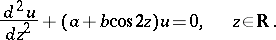It was introduced by E. Mathieu  in the investigation of the oscillations of an elliptic membrane; it is a particular case of a Hill equation.

A fundamental system of solutions of the Mathieu equation has the form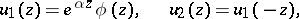(*)

for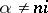,an integer, where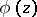is a-periodic function and the Lyapunov characteristic exponentis either real or purely imaginary. For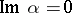one of the solutions grows unboundedly, whereas the other tends to zero as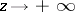(instability zones in the plane of the parameters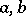); for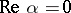these solutions are both bounded (stability zones). On the boundary of these zones (the case excluded in (*)) one of the functions of the fundamental system of solutions is either-periodic or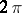-periodic (the latter is called a Mathieu function, cf. Mathieu functions), while the second is obtained from the first through multiplication by. The instability zones have the form of curvilinear triangles with vertices at the points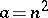,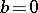,(see , ).

The Mathieu equation is known also in a different form (see ).

How to Cite This Entry:
Mathieu equation. Encyclopedia of Mathematics. URL: http://encyclopediaofmath.org/index.php?title=Mathieu_equation&oldid=47790
This article was adapted from an original article by V.M. Starzhinskii (originator), which appeared in Encyclopedia of Mathematics - ISBN 1402006098. See original article